Next: Improving the evaluation using Up: Mathematical background Previous: Mathematical background   Contents

Using the monotonicity

For a given box we will compute the jacobian matrix using interval analysis. Each row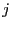of this interval matrix enable to get some information of the corresponding function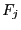.

• if the i-th column of the j-th row is an interval which is strictly negative or strictly positive, thenis monotonic with respect to the unknowns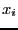• if the i-th column of the j-th row is equal to 0, then functiondoes not depend on the variableIn the first case the minimal and maximal value ofwill be obtained either for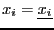or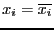and we are able to define the value ofto get successively the minimal and maximal value as we know the sign of the gradient. But this procedure has to be implemented recursively. Indeed we have previously computed the jacobian matrix for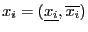but nowhave a fixed value: hence a component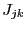of the j-th row which forwas such that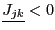and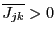may now be a strictly positive or negative intervals. Consequently the minimal and maximal value will be obtained for some combination of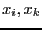in the two sets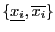and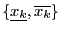. Bus as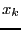has now a fixed value some other component of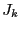may become strictly negative or positive...

The algorithm for computing a sharper evaluation ofis: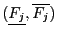=Evaluate(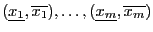)

1. compute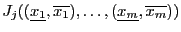2. let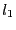be the number of components of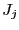such that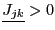or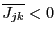and let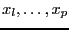be the variables for which this occur
3. if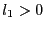loop: for all combination ofin the set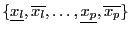:
• if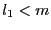• compute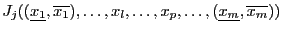• let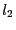be the number of components ofsuch thator• if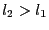, then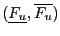=Evaluate(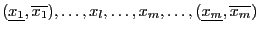)
• otherwise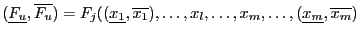)
• if this is the first estimation ofthen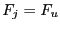• otherwise
• if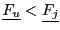, then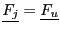• if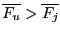, then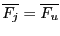• otherwise
•)
• if this is the first estimation ofthen• otherwise
• if, then• if, then4. end loop:
5. otherwise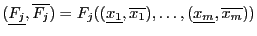6. returnThis procedure has to be repeated for each.Next: Improving the evaluation using Up: Mathematical background Previous: Mathematical background   Contents
Jean-Pierre Merlet 2012-12-20